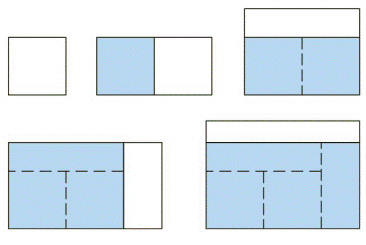Chapter 7.1, Problem 74E

Chapter
Section
Textbook Problem

Let I n = ∫ 0 π / 2 sin n x   d x . (a) Show that I 2 n + 2 ≤ I 2 n + 1 ≤ I 2 n .(b) Use Exercise 50 to show that I 2 n + 2 I 2 n = 2 n + 1 2 n + 2 (c) Use parts (a) and (b) to show that 2 n + 1 2 n + 2 ≤ I 2 n + 1 I 2 n ≤ 1 and deduce that lim n → ∞ I 2 n + 1 / I 2 n = 1. (d) Use part (c) and Exercises 49 and 50 to show that lim n → ∞ 2 1 ⋅ 2 3 ⋅ 4 3 ⋅ 4 5 ⋅ 6 5 ⋅ 6 7 ⋅ ⋅ ⋅ ⋅ ⋅ 2 n 2 n − 1 ⋅ 2 n 2 n + 1 = π 2 This formula is usually written as an infinite product: π 2 = 2 1 ⋅ 2 3 ⋅ 4 3 ⋅ 4 5 ⋅ 6 5 ⋅ 6 7 ⋅ ⋅ ⋅ ⋅ and is called the Wallis product.(e) We construct rectangles as follows. Start with a square of area 1 and attach rectangles of area 1 alternately beside or on top of the previous rectangle (see the figure). Find the limit of the ratios of width to height of these rectangles.To determine

(a)

To show: the relation I2n+2I2n+1I2n

Explanation

A reduction formula can be obtained by using the technique of integration by parts to solve the integral sinnxdx.

Formula used:

Reduction formula is as given below:

sinnxdx=1ncosxsinn1x+n1nsinn2xdx

Given:

The relation to prove and the integral, In=0π2sinnxdx.

Calculation:

Apply the limits of integration in the reduction formula:

0π2sinnxdx=1ncosxsinn1x]0π2+n1n0π2sinn2xdx=1ncosπ2sinn1π2(1ncos0sinn10)+n1n0π2sinn2xdx

Recall that cosπ2=0 and sin0=0. So, the above integral will become:

0π2sinnxdx=1n(0)sinn1π2(1ncos0(0))+n1n0π2sinn2xdx=n1n0π2sinn2xdx

Solve the integral 0π2sin2n+1xdx using the above formula with n as 2n+1. So, substitute for n as 2n+1

0π2sin2n+1xdx=2n+112n+10π2sin2n+12xdx=2n2n+10π2sin2n1xdx …… (1)

Solve the integral 0π2sin2n1xdx again using the formula with n as 2n1

0π2sin2n1xdx=2n112n10π2sin2n12xdx=2n22n10π2sin2n3xdx

Substitute the result for integration in equation (1)

0π2sin2n+1xdx=2n+112n+10π2sin2n+12xdx=2n2n+12n22n10

To determine

(b)

To show: the relation I2n+2I2n=2n+12n+2

To determine

(c)

To show: the relation 2n+12n+2I2n+1I2n1 and limnI2n+2I2n+1=1

To determine

(d)

To show: the relation limn2123434565672n2n12n2n+1=π2

To determine

(e)

To find: the limit of ratios of the width to height of the rectangles

Still sussing out bartleby?

Check out a sample textbook solution.

See a sample solution

The Solution to Your Study Problems

Bartleby provides explanations to thousands of textbook problems written by our experts, many with advanced degrees!

Get Started

Find the domain of each function. 19. (a) f(x)=1ex21e1x2 (b) f(x)=1+xecosx

Single Variable Calculus: Early Transcendentals, Volume I

Find the x- and y-intercepts of the graph of y=x22x3.

Calculus: An Applied Approach (MindTap Course List)

Define the terms population and sample, and explain the role of each in a research study.

Essentials of Statistics for The Behavioral Sciences (MindTap Course List)

In Problems 1-6, find the second derivative.

Mathematical Applications for the Management, Life, and Social Sciences

True or False: converges conditionally.

Study Guide for Stewart's Single Variable Calculus: Early Transcendentals, 8th

In Exercises 110, find the graphical solution of each inequality. 5x3y15

Finite Mathematics for the Managerial, Life, and Social Sciences